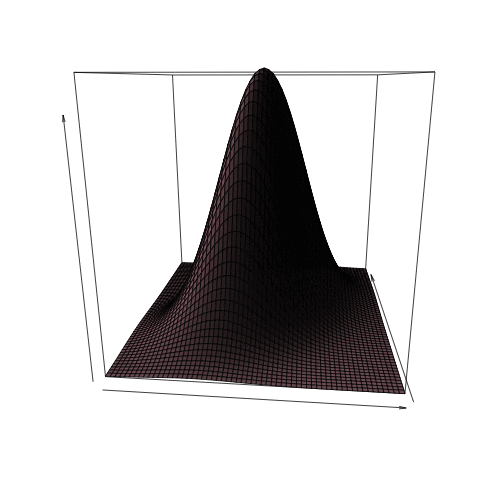[This article was first published on Freakonometrics - Tag - R-english, and kindly contributed to R-bloggers]. (You can report issue about the content on this page here)
Want to share your content on R-bloggers? click here if you have a blog, or here if you don't.

Last week, we’ve introduced the concept of exchangeable variables, i.e. satisfyingfor any matrix, i.e.is a permutation matrix:belongs to the orthogonal group,, and with elements in. It is possible to extend that family, considering all matrices in the orthogonal group, i.e.for all. Since orthogonal matrices can be seen as rotation matrices,it will mean, e.g. that density is invariant by rotations,So level curves will be circles (in dimension 2), or more generally spheres. This will yield the concept of spherical distribution (or spherically contoured distributions), that will be extended to elliptical distributions (see e.g. Hartman & Wintner (1940), Kelker (1970) or Cambanis, Huang & Simons (1979))
• spherically contoured distributionshas a spherical distribution if its characteristic function can be writtenfor some function. Such a function will be called generator, and we say that. Equivalently,has a spherical distribution if. A popular example is the Gaussian distribution (centered, with independent margins)Note that there exist a nice stochastic representation of spherically contoured distribution,whereis a positive random variable, independent of, uniformly distributed over the unit sphere of, i.e.This construction can be related to the following decomposition• from circles to ellipses
From that geometric interpretation, and from the connexion with the Gaussian distribution, it is possible to move circles (and spheres) to ellipses. Ifandfor some variance-covariance matrix, thenwhereis some square-root of, i.e.. Based on that transformation (through that covariance matrix), level curves of the density are non longer circles, be ellipses,if we change only the variance of the first component (above), while if we change the variance of the second one (below)If we change only the correlation, the axis of the ellipse are still the first and the second diagonalwhile the impact of correlation when X and Y do not have the same variance gives us the following transformations,• elliptically contoured distributions
A random vector is said to be elliptical,ifwhereis some square-root of, i.e., and. It characteristic function is thenFurther, in that case,while.Note that the two most popular elliptical distributions (the Gaussian and Student’s t) can be obtained in R as follows,
```> library(mnormt)
> x <- seq(-2,4,length=21)
> mu <- c(1,3,2)
> Sigma <- matrix(c(1,2,0,2,5,0.5,0,0.5,3), 3, 3)
> df <- 4
> x=c(0,0);y=c(0,1); z=c(0,2)
> dmt(cbind(x,y,z), mu, Sigma,df)
 0.006957689 0.020602030
> rmt(n=5, mu, Sigma, df)
[,1]       [,2]      [,3]
[1,]  0.42210352  2.7539135  1.659392
[2,]  1.07968146 -0.1364883  4.851956
[3,] -0.04107115  1.6163407  4.123731
[4,]  0.19784451  2.9329165  1.013374
[5,]  1.13456027  0.4737548 -2.054909```

To leave a comment for the author, please follow the link and comment on their blog: Freakonometrics - Tag - R-english.

R-bloggers.com offers daily e-mail updates about R news and tutorials about learning R and many other topics. Click here if you're looking to post or find an R/data-science job.
Want to share your content on R-bloggers? click here if you have a blog, or here if you don't.

# Never miss an update! Subscribe to R-bloggers to receive e-mails with the latest R posts.(You will not see this message again.)

Click here to close (This popup will not appear again)